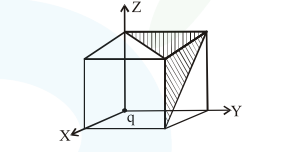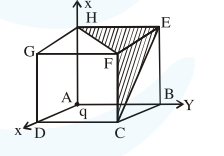# A charge ' q' is placed at one corner of a cube`
Question:

A charge ' $q$ ' is placed at one corner of a cube as shown in figure.The flux of electrostatic field $\vec{E}$ through the shaded area is:1. $\frac{q}{4 \varepsilon_{0}}$

2. $\frac{q}{24 \varepsilon_{0}}$

3. $\frac{q}{48 \varepsilon_{0}}$

4. $\frac{q}{8 \varepsilon_{0}}$

Correct Option: , 2

Solution:flux through cube $=\frac{\mathrm{q}}{8 \epsilon_{0}}$

flux through surfaces $\mathrm{ABEH}, \mathrm{ADGH}, \mathrm{ABCD}$ will be zero

$\phi(\mathrm{EFGH})=\phi(\mathrm{DCFG})=\phi(\mathrm{EBCF})=\frac{1}{3}\left(\frac{\mathrm{q}}{8 \epsilon_{0}}\right)$

$=\frac{\mathrm{q}}{24 \epsilon_{0}}$